Linear Regression and Correlation

# 69 Testing the Significance of the Correlation Coefficient

The correlation coefficient, r, tells us about the strength and direction of the linear relationship between X1 and X2.

The sample data are used to compute r, the correlation coefficient for the sample. If we had data for the entire population, we could find the population correlation coefficient. But because we have only sample data, we cannot calculate the population correlation coefficient. The sample correlation coefficient, r, is our estimate of the unknown population correlation coefficient.

• ρ = population correlation coefficient (unknown)
• r = sample correlation coefficient (known; calculated from sample data)

The hypothesis test lets us decide whether the value of the population correlation coefficient ρ is “close to zero” or “significantly different from zero”. We decide this based on the sample correlation coefficient r and the sample size n.

If the test concludes that the correlation coefficient is significantly different from zero, we say that the correlation coefficient is “significant.”

• Conclusion: There is sufficient evidence to conclude that there is a significant linear relationship between X1 and X2 because the correlation coefficient is significantly different from zero.
• What the conclusion means: There is a significant linear relationship X1 and X2. If the test concludes that the correlation coefficient is not significantly different from zero (it is close to zero), we say that correlation coefficient is “not significant”.

### Performing the Hypothesis Test

• Null Hypothesis: H0: ρ = 0
• Alternate Hypothesis: Ha: ρ ≠ 0
What the Hypotheses Mean in Words
• Null Hypothesis H0: The population correlation coefficient IS NOT significantly different from zero. There IS NOT a significant linear relationship (correlation) between X1 and X2 in the population.
• Alternate Hypothesis Ha: The population correlation coefficient is significantly different from zero. There is a significant linear relationship (correlation) between X1 and X2 in the population.

Drawing a ConclusionThere are two methods of making the decision concerning the hypothesis. The test statistic to test this hypothesis is: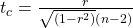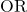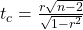Where the second formula is an equivalent form of the test statistic, n is the sample size and the degrees of freedom are n-2. This is a t-statistic and operates in the same way as other t tests. Calculate the t-value and compare that with the critical value from the t-table at the appropriate degrees of freedom and the level of confidence you wish to maintain. If the calculated value is in the tail then cannot accept the null hypothesis that there is no linear relationship between these two independent random variables. If the calculated t-value is NOT in the tailed then cannot reject the null hypothesis that there is no linear relationship between the two variables.

A quick shorthand way to test correlations is the relationship between the sample size and the correlation. If: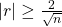then this implies that the correlation between the two variables demonstrates that a linear relationship exists and is statistically significant at approximately the 0.05 level of significance. As the formula indicates, there is an inverse relationship between the sample size and the required correlation for significance of a linear relationship. With only 10 observations, the required correlation for significance is 0.6325, for 30 observations the required correlation for significance decreases to 0.3651 and at 100 observations the required level is only 0.2000.

Correlations may be helpful in visualizing the data, but are not appropriately used to “explain” a relationship between two variables. Perhaps no single statistic is more misused than the correlation coefficient. Citing correlations between health conditions and everything from place of residence to eye color have the effect of implying a cause and effect relationship. This simply cannot be accomplished with a correlation coefficient. The correlation coefficient is, of course, innocent of this misinterpretation. It is the duty of the analyst to use a statistic that is designed to test for cause and effect relationships and report only those results if they are intending to make such a claim. The problem is that passing this more rigorous test is difficult so lazy and/or unscrupulous “researchers” fall back on correlations when they cannot make their case legitimately.

Define a t Test of a Regression Coefficient, and give a unique example of its use.

Definition:

A t test is obtained by dividing a regression coefficient by its standard error and then comparing the result to critical values for Students’ t with Error df. It provides a test of the claim that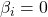when all other variables have been included in the relevant regression model.

Example:

Suppose that 4 variables are suspected of influencing some response. Suppose that the results of fitting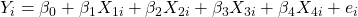include:

 Variable Regression coefficient Standard error of regular coefficient .5 1 -3 .4 2 +2 .02 3 +1 .6 4 -.5

t calculated for variables 1, 2, and 3 would be 5 or larger in absolute value while that for variable 4 would be less than 1. For most significance levels, the hypothesis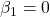would be rejected. But, notice that this is for the case when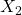,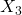, and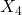have been included in the regression. For most significance levels, the hypothesis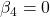would be continued (retained) for the case where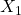,, andare in the regression. Often this pattern of results will result in computing another regression involving only,,, and examination of the t ratios produced for that case.

The correlation between scores on a neuroticism test and scores on an anxiety test is high and positive; therefore

1. anxiety causes neuroticism
2. those who score low on one test tend to score high on the other.
3. those who score low on one test tend to score low on the other.
4. no prediction from one test to the other can be meaningfully made.

c. those who score low on one test tend to score low on the other.